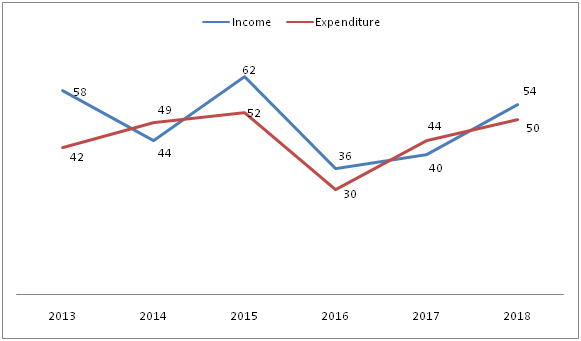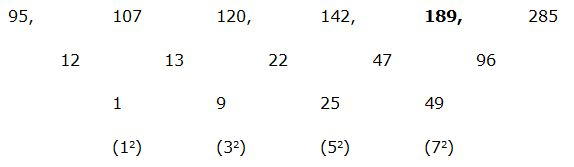# SBI Clerk prelims Quantitative Aptitude Questions 2019 (Day-05)

Quantitative aptitude is one of the most focusing subjects among banking aspirants.In SBI Clerk Prelims there are 35 Marks allotted for this section. The Marks itself shows how weightage has given for Quantitative aptitude section. The SBI Clerk 2019 exam is approaching very shortly. The exam tentatively scheduled in June 2019. Understand the importance of time and use it in the right way. As we know that SBI aptitude test questions are easy but difficult to solve within the given time limit. So practice the only solution to perform well at the time of examination. Keeping all the things in mind we are giving attention for SBI Clerk Prelims Quantitative Aptitude section. Here you can get SBI Clerk Prelims Quantitative Aptitude Questions and answer on a daily basis. SBI Clerk Prelims Quantitative Aptitude Quiz will enhance your sped and accuracy in a self-directed way.

[WpProQuiz 6197]

Directions (Q. 1 – 5): Find the wrong term in the following number series?

1) 95, 107, 120, 142, 190, 285

a) 95

b) 107

c) 142

d) 190

e) 285

2) 14, 15, 32, 97, 400, 2005

a) 97

b) 32

c) 400

d) 2005

e) 15

3) 69984, 11664, 1944, 322, 54, 9

a) 11664

b) 54

c) 1944

d) 69984

e) 322

4) 72, 36, 54, 136, 472.5, 2126.25

a) 54

b) 136

c) 472.5

d) 2126.25

e) 72

5) 12, 34, 100, 296, 892, 2674

a) 34

b) 892

c) 296

d) 100

e) 2674

Directions (Q. 6 – 10): Study the following information carefully and answer the given questions.

The following line graph shows the Income and Expenditure (In thousands) of a company over the years.6) The profit/loss made by the company in the year 2014 is approximately what percentage of the profit/loss made by the company in the year 2018?

a) 125 %

b) 140 %

c) 105 %

d) 95 %

e) 80 %

7) In which year, the profit/loss is third lowest in the company?

a) 2014

b) 2015

c) 2018

d) 2016

e) None of these

8) Find the average income of the company in all the given years together?

a) Rs. 42000

b) Rs. 49000

c) Rs. 53000

d) Rs. 55000

e) None of these

9) The income of the company in the year 2013, 2015 and 2017 together is approximately what percentage of the expenditure of the company in the year 2014, 2016 and 2018 together?

a) 150 %

b) 165 %

c) 125 %

d) 180 %

e) 105 %

10) Find the difference between the average income to that of average expenditure of the company?

a) Rs. 4500

b) Rs. 5500

c) Rs. 3000

d) Rs. 6000

e) None of these

Directions (Q. 1 – 5):

The correct series is,The difference of difference is, 12, 32, 52, 72,….

The wrong term is, 190

The correct series is,

14, 15, 32, 99, 400, 2005

The pattern is, *1 + 1, *2 + 2, *3 + 3, *4 + 4, *5 + 5,….

The wrong term is, 97

The correct series is,

69984, 11664, 1944, 324, 54, 9

The pattern is, ÷ 6

The wrong term is, 322

The correct series is,

72, 36, 54, 135, 472.5, 2126.25

The pattern is, *0.5, *1.5, *2.5, *3.5, *4.5,….

The wrong term is, 136

The correct series is,

12, 34, 100, 298, 892, 2674

The pattern is, *3 – 2

The wrong term is, 296

Directions (Q. 6 – 10):

The profit/loss made by the company in the year 2014

= > (49000 – 44000) = Rs. 5000 loss

The profit/loss made by the company in the year 2018

= > (54000 – 50000) = Rs. 4000 profit

Required % = (5000/4000)*100 = 125 %

In 2013, Profit = 58000 – 42000 = Rs. 16000

In 2014, Loss = 49000 – 44000 = Rs. 5000

In 2015, Profit = 62000 – 52000 = Rs. 10000

In 2016, Profit = 36000 – 30000 = Rs. 6000

In 2017, Loss = 44000 – 40000 = Rs. 4000

In 2018, Profit = 54000 – 50000 = Rs. 4000

The company has third lowest number of profit/loss in the year 2016.

The average income of the company in all the given years together

= > (58000 + 44000 + 62000 + 36000 + 40000 + 54000)/6

= > 294000/6 = Rs. 49000

The income of the company in the year 2013, 2015 and 2017 together

= > 58000 + 62000 + 40000 = Rs. 160000

The expenditure of the company in the year 2014, 2016 and 2018 together

= > 49000 + 30000 + 50000 = Rs. 129000

Required % = (160000/129000)*100 = 124 % = 125 %

The average income of the company

= > (58000 + 44000 + 62000 + 36000 + 40000 + 54000)/6

= > 294000/6 = Rs. 49000

The average expenditure of the company

= > (42000 + 49000 + 52000 + 30000 + 44000 + 50000)/6

= > 267000/6 = Rs. 44500

Required difference = 49000 – 44500 = Rs. 4500